# TrigonometryThe Canadarm2 robotic manipulator on the International Space Station is operated by controlling the angles of its joints. Calculating the final position of the astronaut at the end of the arm requires repeated use of the trigonometric functions of those angles.

Trigonometry (from Greek Τριγωνομετρία "tri = three" + "gon = angle" + "metr[y] = to measure") is a branch of mathematics that deals with triangles, particularly those plane triangles in which one angle has 90 degrees (right triangles). Trigonometry deals with relationships between the sides and the angles of triangles and with the trigonometric functions, which describe those relationships.

Trigonometry has applications in both pure mathematics and in applied mathematics, where it is essential in many branches of science and technology. It is usually taught in secondary schools either as a separate course or as part of a precalculus course. Trigonometry is informally called "trig" or "trigo."

A branch of trigonometry, called spherical trigonometry, studies triangles on spheres, and is important in astronomy and navigation.

## History

Trigonometry was developed for use in sailing as a navigation method used with astronomy. The origins of trigonometry can be traced to the civilizations of ancient Egypt, Mesopotamia and the Indus Valley (India), more than 4000 years ago. The common practice of measuring angles in degrees, minutes and seconds comes from the Babylonian's base sixty system of numeration.

The first recorded use of trigonometry came from the Hellenistic mathematician Hipparchus c. 150 B.C.E., who compiled a trigonometric table using the sine for solving triangles. Ptolemy further developed trigonometric calculations c. 100 C.E.

The ancient Sinhalese in Sri Lanka, when constructing reservoirs in the Anuradhapura kingdom, used trigonometry to calculate the gradient of the water flow. Archeological research also provides evidence of trigonometry used in other unique hydrological structures dating back to 4 B.C.E.

The Indian mathematician Aryabhata in 499, gave tables of half chords which are now known as sine tables, along with cosine tables. He used zya for sine, kotizya for cosine, and otkram zya for inverse sine, and also introduced the versine. Another Indian mathematician, Brahmagupta in 628, used an interpolation formula to compute values of sines, up to the second order of the Newton-Stirling interpolation formula.

In the tenth century, the Persian mathematician and astronomer Abul Wáfa introduced the tangent function and improved methods of calculating trigonometry tables. He established the angle addition identities, for example, sin (a + b), and discovered the sine formula for spherical geometry: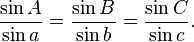$\frac{\sin A}{\sin a} = \frac{\sin B}{\sin b} = \frac{\sin C}{\sin c}.$

Also in the late tenth and early eleventh centuries, the Egyptian astronomer Ibn Yunus performed many careful trigonometric calculations and demonstrated the formula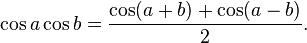$\cos a \cos b = \frac{\cos(a+b) + \cos(a-b)}{2}.$

Persian mathematician Omar Khayyám (1048-1131) combined trigonometry and approximation theory to provide methods of solving algebraic equations by geometrical means. Khayyam solved the cubic equation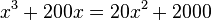$x^3 + 200 x = 20 x^2 + 2000$ and found a positive root of this cubic by considering the intersection of a rectangular hyperbola and a circle. An approximate numerical solution was then found by interpolation in trigonometric tables.

Detailed methods for constructing a table of sines for any angle were given by the Indian mathematician Bhaskara in 1150, along with some sine and cosine formulae. Bhaskara also developed spherical trigonometry.

The thirteenth century Persian mathematician Nasir al-Din Tusi, along with Bhaskara, was probably the first to treat trigonometry as a distinct mathematical discipline. Nasir al-Din Tusi in his Treatise on the Quadrilateral was the first to list the six distinct cases of a right angled triangle in spherical trigonometry.

In the fourteenth century, Persian mathematician al-Kashi and Timurid mathematician Ulugh Beg (grandson of Timur) produced tables of trigonometric functions as part of their studies of astronomy.

The mathematician Bartholemaeus Pitiscus published an influential work on trigonometry in 1595 which may have coined the word "trigonometry" itself.

## Overview

If one angle of a triangle is 90 degrees and one of the other angles is known, the third is thereby fixed, because the three angles of any triangle add up to 180 degrees. The two acute angles therefore add up to 90 degrees: They are complementary angles. The shape of a right triangle is completely determined, up to similarity, by the angles. This means that once one of the other angles is known, the ratios of the various sides are always the same regardless of the overall size of the triangle. These ratios are given by the following trigonometric functions of the known angle A, where a, b, and c refer to the lengths of the sides in the accompanying figure:

• The sine function (sin), defined as the ratio of the side opposite the angle to the hypotenuse.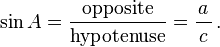$\sin A=\frac{\textrm{opposite}}{\textrm{hypotenuse}}=\frac{a}{\,c\,}\,.$
• The cosine function (cos), defined as the ratio of the adjacent leg to the hypotenuse.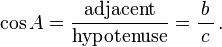$\cos A=\frac{\textrm{adjacent}}{\textrm{hypotenuse}}=\frac{b}{\,c\,}\,.$
• The tangent function (tan), defined as the ratio of the opposite leg to the adjacent leg.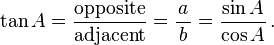$\tan A=\frac{\textrm{opposite}}{\textrm{adjacent}}=\frac{a}{\,b\,}=\frac{\sin A}{\cos A}\,.$

The hypotenuse is the side opposite to the 90 degree angle in a right triangle; it is the longest side of the triangle, and one of the two sides adjacent to angle A. The adjacent leg is the other side that is adjacent to angle A. The opposite side is the side that is opposite to angle A. The terms perpendicular and base are sometimes used for the opposite and adjacent sides respectively. Many people find it easy to remember what sides of the right triangle are equal to sine, cosine, or tangent, by memorizing the word SOH-CAH-TOA (see below under Mnemonics).

The reciprocals of these functions are named the cosecant (csc or cosec), secant (sec) and cotangent (cot), respectively. The inverse functions are called the arcsine, arccosine, and arctangent, respectively. There are arithmetic relations between these functions, which are known as trigonometric identities.

With these functions one can answer virtually all questions about arbitrary triangles by using the law of sines and the law of cosines. These laws can be used to compute the remaining angles and sides of any triangle as soon as two sides and an angle or two angles and a side or three sides are known. These laws are useful in all branches of geometry, since every polygon may be described as a finite combination of triangles.

### Extending the definitions

The above definitions apply to angles between 0 and 90 degrees (0 and π/2 radians) only. Using the unit circle, one can extend them to all positive and negative arguments (see trigonometric function). The trigonometric functions are periodic, with a period of 360 degrees or 2π radians. That means their values repeat at those intervals.

The trigonometric functions can be defined in other ways besides the geometrical definitions above, using tools from calculus and infinite series. With these definitions the trigonometric functions can be defined for complex numbers. The complex function cis is particularly useful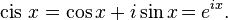$\operatorname{cis}\,x = \cos x + i\sin x \! = e^{ix}.$

See Euler's and De Moivre's formulas.

### Mnemonics

Students often use mnemonics to remember facts and relationships in trigonometry. For example, the sine, cosine, and tangent ratios in a right triangle can be remembered by representing them as strings of letters, as in SOH-CAH-TOA.

Sine = Opposite ÷ Hypotenuse

Alternatively, one can devise sentences which consist of words beginning with the letters to be remembered. For example, to recall that Tan = Opposite/Adjacent, the letters T-O-A must be remembered. Any memorable phrase constructed of words beginning with the letters T-O-A will serve.

It is of ethnographic interest to note that the mnemonic TOA-CAH-SOH can be translated in the local Singaporean Hokkien dialect to "big-legged woman," serving as an additional learning aid for students in Singapore..

Another type of mnemonic describes facts in a simple, memorable way, such as "Plus to the right, minus to the left; positive height, negative depth," which refers to trigonometric functions generated by a revolving line.

### Calculating trigonometric functions

Trigonometric functions were among the earliest uses for mathematical tables. Such tables were incorporated into mathematics textbooks and students were taught to look up values and how to interpolate between the values listed to get higher accuracy. Slide rules had special scales for trigonometric functions.

Today scientific calculators have buttons for calculating the main trigonometric functions (sin, cos, tan and sometimes cis) and their inverses. Most allow a choice of angle measurement methods, degrees, radians and, sometimes, Grad. Most computer programming languages provide function libraries that include the trigonometric functions. The floating point unit hardware incorporated into the microprocessor chips used in most personal computers have built in instructions for calculating trigonometric functions.

## Applications of trigonometry

There are an enormous number of applications of trigonometry and trigonometric functions. For instance, the technique of triangulation is used in astronomy to measure the distance to nearby stars, in geography to measure distances between landmarks, and in satellite navigation systems. The sine and cosine functions are fundamental to the theory of periodic functions such as those that describe sound and light waves.

Fields which make use of trigonometry or trigonometric functions include astronomy (especially, for locating the apparent positions of celestial objects, in which spherical trigonometry is essential) and hence navigation (on the oceans, in aircraft, and in space), music theory, acoustics, optics, analysis of financial markets, electronics, probability theory, statistics, biology, medical imaging (CAT scans and ultrasound), pharmacy, chemistry, number theory (and hence cryptology), seismology, meteorology, oceanography, many physical sciences, land surveying and geodesy, architecture, phonetics, economics, electrical engineering, mechanical engineering, civil engineering, computer graphics, cartography, crystallography, and game development.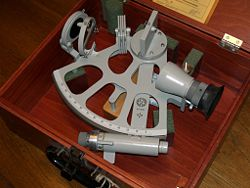Marine sextants like this are used to measure the angle of the sun or stars with respect to the horizon. Using trigonometry and a marine chronometer, the position of the ship can then be determined from several such measurements.

## Common formulae

Certain equations involving trigonometric functions are true for all angles and are known as trigonometric identities. Many express important geometric relationships. For example, the Pythagorean identities are an expression of the Pythagorean Theorem. Here are some of the more commonly used identities, as well as the most important formulae connecting angles and sides of an arbitrary triangle. For more identities see trigonometric identity.

### Trigonometric identities

#### Pythagorean identities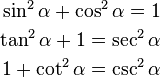\begin{align} \sin^2 \alpha + \cos^2 \alpha = 1 \\ \tan^2 \alpha + 1 = \sec^2 \alpha \\ 1+\cot^2 \alpha = \csc^2 \alpha \end{align}

#### Sum and product identities

##### Sum to product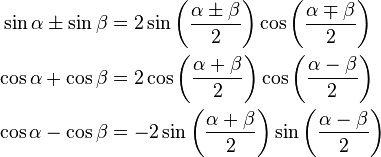\begin{align} \sin \alpha \pm \sin \beta &= 2\sin \left( \frac{\alpha \pm \beta}{2}\right)\cos \left(\frac{\alpha \mp \beta}{2} \right)\\ \cos \alpha + \cos \beta &= 2\cos \left(\frac{\alpha + \beta}{2} \right)\cos \left(\frac{\alpha - \beta}{2}\right)\\ \cos \alpha - \cos \beta &= -2\sin \left(\frac{\alpha + \beta}{2} \right) \sin \left(\frac{\alpha - \beta}{2}\right) \end{align}
##### Product to sum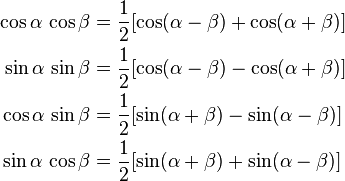\begin{align} \cos \alpha \,\cos \beta &= \frac{1}{2}[\cos(\alpha - \beta) + \cos (\alpha + \beta)]\\ \sin \alpha \,\sin \beta &= \frac{1}{2}[\cos(\alpha - \beta) - \cos (\alpha + \beta)]\\ \cos \alpha \,\sin \beta &= \frac{1}{2}[\sin(\alpha + \beta) - \sin (\alpha - \beta)]\\ \sin \alpha \,\cos \beta &= \frac{1}{2}[\sin(\alpha + \beta) + \sin (\alpha - \beta)] \end{align}
##### Sine, cosine, and tangent of a sum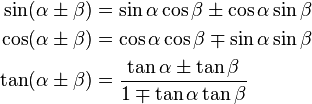\begin{align} \sin(\alpha \pm \beta) &= \sin \alpha \cos \beta \pm \cos \alpha \sin \beta \\ \cos(\alpha \pm \beta) &= \cos \alpha \cos \beta \mp \sin \alpha \sin \beta \\ \tan(\alpha \pm \beta) &= \frac{\tan \alpha \pm \tan \beta}{1 \mp \tan \alpha \tan \beta} \end{align}

#### Half-angle identities

Note that$\pm$ is correct, it means it may be either one, depending on the value of A/2.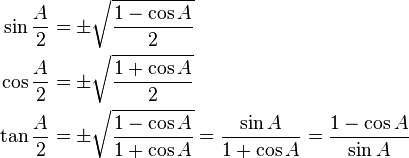\begin{align} \sin \frac{A}{2} &= \pm \sqrt{\frac{1-\cos A}{2}} \\ \cos \frac{A}{2} &= \pm \sqrt{\frac{1+\cos A}{2}} \\\tan \frac{A}{2} &= \pm \sqrt{\frac{1-\cos A}{1+\cos A}} = \frac {\sin A}{1+\cos A} = \frac {1-\cos A}{\sin A} \end{align}

#### Stereographic (or parametric) identities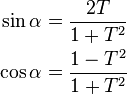\begin{align} \sin \alpha &= \frac{2T}{1+T^2} \\ \cos \alpha &= \frac{1-T^2}{1+T^2}\\ \end{align}

where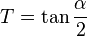$T=\tan \frac{\alpha}{2}$.

### Triangle identitiesLaws of Sines and Cosines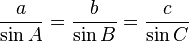$\frac{a}{\sin A}=\frac{b}{\sin B}=\frac{c}{\sin C}$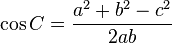$\cos C=\frac{a^2+b^2-c^2}{2ab}$

In the following identities, A, B, and C are the angles of a triangle and a, b, and c are the lengths of sides of the triangle opposite the respective angles.

#### Law of sines

The law of sines (also know as the "sine rule") for an arbitrary triangle states:$\frac{a}{\sin A} = \frac{b}{\sin B} = \frac{c}{\sin C} = 2R,$

where R is the radius of the circumcircle of the triangle.

#### Law of cosines

The law of cosines (also known as the cosine formula, or the "cos rule") is an extension of the Pythagorean theorem to arbitrary triangles: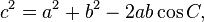$c^2=a^2+b^2-2ab\cos C ,\,$

or equivalently: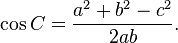$\cos C=\frac{a^2+b^2-c^2}{2ab}.\,$

#### Law of tangents

The law of tangents:$\frac{a+b}{a-b}=\frac{\tan\left[\tfrac{1}{2}(A+B)\right]}{\tan\left[\tfrac{1}{2}(A-B)\right]}$